# Best Pandas Tutorial | Learn with 50 Examples

Pandas being one of the most popular package in Python is widely used for data manipulation. It is a very powerful and versatile package which makes data cleaning and wrangling much easier and pleasant.
The Pandas library has a great contribution to the python community and it makes python as one of the top programming language for data science and analytics. It has become first choice of data analysts and scientists for data analysis and manipulation.Data Analysis with Python : Pandas Step by Step Guide
What is pandas package?
Pandas package has many functions which are the essence for data handling and manipulation. In short, it can perform the following tasks for you -
1. Create a structured data set similar to R's data frame and Excel spreadsheet.
2. Reading data from various sources such as CSV, TXT, XLSX, SQL database, R etc.
3. Selecting particular rows or columns from data set
4. Arranging data in ascending or descending order
5. Filtering data based on some conditions
6. Summarizing data by classification variable
7. Reshape data into wide or long format
8. Time series analysis
9. Merging and concatenating two datasets
10. Iterate over the rows of dataset
11. Writing or Exporting data in CSV or Excel format
Datasets:
In this tutorial we will use two datasets: 'income' and 'iris'.
1. 'income' data : This data contains the income of various states from 2002 to 2015. The dataset contains 51 observations and 16 variables. Download link
2. 'iris' data: It comprises of 150 observations with 5 variables. We have 3 species of flowers(50 flowers for each specie) and for all of them the sepal length and width and petal length and width are given. Download link

Important pandas functions to remember
The following is a list of common tasks along with pandas functions.
Utility Functions
Extract Column Names df.columns
Select first 2 rows df.iloc[:2]
Select first 2 columns df.iloc[:,:2]
Select columns by name df.loc[:,["col1","col2"]]
Select random no. of rows df.sample(n = 10)
Select fraction of random rows df.sample(frac = 0.2)
Rename the variables df.rename( )
Selecting a column as index df.set_index( )
Removing rows or columns df.drop( )
Sorting values df.sort_values( )
Grouping variables df.groupby( )
Filtering df.query( )
Finding the missing values df.isnull( )
Dropping the missing values df.dropna( )
Removing the duplicates df.drop_duplicates( )
Creating dummies pd.get_dummies( )
Ranking df.rank( )
Cumulative sum df.cumsum( )
Quantiles df.quantile( )
Selecting numeric variables df.select_dtypes( )
Concatenating two dataframes pd.concat()
Merging on basis of common variable pd.merge( )
Importing pandas library
You need to import or load the Pandas library first in order to use it. By "Importing a library", it means loading it into the memory and then you can use it. Run the following code to import pandas library:
`import pandas as pd`
The "pd" is an alias or abbreviation which will be used as a shortcut to access or call pandas functions. To access the functions from pandas library, you just need to type pd.function instead of  pandas.function every time you need to apply it.
Importing Dataset
To read or import data from CSV file, you can use read_csv() function. In the function, you need to specify the file location of your CSV file.
`income = pd.read_csv("C:\\Users\\Hp\\Python\\Basics\\income.csv")`
``` Index       State    Y2002    Y2003    Y2004    Y2005    Y2006    Y2007  \
0     A     Alabama  1296530  1317711  1118631  1492583  1107408  1440134
1     A      Alaska  1170302  1960378  1818085  1447852  1861639  1465841
2     A     Arizona  1742027  1968140  1377583  1782199  1102568  1109382
3     A    Arkansas  1485531  1994927  1119299  1947979  1669191  1801213
4     C  California  1685349  1675807  1889570  1480280  1735069  1812546
Y2008    Y2009    Y2010    Y2011    Y2012    Y2013    Y2014    Y2015
0  1945229  1944173  1237582  1440756  1186741  1852841  1558906  1916661
1  1551826  1436541  1629616  1230866  1512804  1985302  1580394  1979143
2  1752886  1554330  1300521  1130709  1907284  1363279  1525866  1647724
3  1188104  1628980  1669295  1928238  1216675  1591896  1360959  1329341
4  1487315  1663809  1624509  1639670  1921845  1156536  1388461  1644607
```
Get Variable Names
By using `income.columns`command, you can fetch the names of variables of a data frame.
```Index(['Index', 'State', 'Y2002', 'Y2003', 'Y2004', 'Y2005', 'Y2006', 'Y2007',
'Y2008', 'Y2009', 'Y2010', 'Y2011', 'Y2012', 'Y2013', 'Y2014', 'Y2015'],
dtype='object')
```
`income.columns[0:2]` returns first two column names 'Index', 'State'. In python, indexing starts from 0.
Knowing the Variable types
You can use the dataFrameName.dtypes command to extract the information of types of variables stored in the data frame.
`income.dtypes `
```Index    object
State    object
Y2002     int64
Y2003     int64
Y2004     int64
Y2005     int64
Y2006     int64
Y2007     int64
Y2008     int64
Y2009     int64
Y2010     int64
Y2011     int64
Y2012     int64
Y2013     int64
Y2014     int64
Y2015     int64
dtype: object
```
Here 'object' means strings or character variables. 'int64' refers to numeric variables (without decimals).

To see the variable type of one variable (let's say "State") instead of all the variables, you can use the command below -
`income['State'].dtypes`
It returns dtype('O'). In this case, 'O' refers to object i.e. type of variable as character.
Changing the data types
Y2008 is an integer. Suppose we want to convert it to float (numeric variable with decimals) we can write:
```income.Y2008 = income.Y2008.astype(float)
income.dtypes```
```Index     object
State     object
Y2002      int64
Y2003      int64
Y2004      int64
Y2005      int64
Y2006      int64
Y2007      int64
Y2008    float64
Y2009      int64
Y2010      int64
Y2011      int64
Y2012      int64
Y2013      int64
Y2014      int64
Y2015      int64
dtype: object```
To view the dimensions or shape of the data
`income.shape`
``` (51, 16)
```
51 is the number of rows and 16 is the number of columns.

You can also use shape to see the number of rows (similar to nrow() in R) and shape for number of columns (similar to ncol() in R).
```income.shape
income.shape```
To view only some of the rows
By default head( ) shows first 5 rows. If we want to see a specific number of rows we can mention it in the parenthesis. Similarly tail( ) function shows last 5 rows by default.
```income.head()
income.tail()
income.tail(2)  #shows last 2 rows```

Alternatively, any of the following commands can be used to fetch first five rows.
`income[0:5] `
`income.iloc[0:5]`
Define Categorical Variable
Like factors() function in R, we can include categorical variable in python using "category" dtype.
```s = pd.Series([1,2,3,1,2], dtype="category")
s```
```0    1
1    2
2    3
3    1
4    2
dtype: category
Categories (3, int64): [1, 2, 3]
```
Extract Unique Values
The unique() function shows the unique levels or categories in the dataset.
`income.Index.unique()`
```array(['A', 'C', 'D', ..., 'U', 'V', 'W'], dtype=object)
```

The nunique( ) shows the number of unique values.
`income.Index.nunique()`
It returns 19 as index column contains distinct 19 values.
Generate Cross Tab
pd.crosstab( ) is used to create a bivariate frequency distribution. Here the bivariate frequency distribution is between Index and State columns.
`pd.crosstab(income.Index,income.State)`
Creating a frequency distribution
income.Index selects the 'Index' column of 'income' dataset and value_counts( ) creates a frequency distribution. By default ascending = False i.e. it will show the 'Index' having the maximum frequency on the top.
`income.Index.value_counts(ascending = True) `
```F    1
G    1
U    1
L    1
H    1
P    1
R    1
D    2
T    2
S    2
V    2
K    2
O    3
C    3
I    4
W    4
A    4
M    8
N    8
Name: Index, dtype: int64
```
To draw the samples
income.sample( ) is used to draw random samples from the dataset containing all the columns. Here n = 5 depicts we need 5 columns and frac = 0.1 tells that we need 10 percent of the data as my sample.
```income.sample(n = 5)
income.sample(frac = 0.1)```
Selecting only a few of the columns
There are multiple ways you can select a particular column. Both the following line of code selects State variable from income data frame.
```income["State"]
income.State
```
To select multiple columns by name, you can use the following syntax.
`income[["Index","State","Y2008"]]`
To select only specific columns and rows, we use either loc[ ] or iloc[ ] functions. The index or columns to be selected are passed as lists. "Index":"Y2008" denotes the that all the columns from Index to Y2008 are to be selected.
Syntax of df.loc[  ]
`df.loc[row_index , column_index]`
```income.loc[:,["Index","State","Y2008"]]
income.loc[0:2,["Index","State","Y2008"]]  #Selecting rows with Index label 0 to 2 & columns
income.loc[:,"Index":"Y2008"]  #Selecting consecutive columns
#In the above command both Index and Y2008 are included.
income.iloc[:,0:5]  #Columns from 1 to 5 are included. 6th column not included```
Difference between loc and iloc
loc considers rows (or columns) with particular labels from the index. Whereas iloc considers rows (or columns) at particular positions in the index so it only takes integers.
`x = pd.DataFrame({"var1" : np.arange(1,20,2)}, index=[9,8,7,6,10, 1, 2, 3, 4, 5])`
```    var1
9      1
8      3
7      5
6      7
10     9
1     11
2     13
3     15
4     17
5     19
```

iloc Code

```x.iloc[:3]
Output:
var1
9     1
8     3
7     5
```

loc code

```x.loc[:3]
Output:
var1
9      1
8      3
7      5
6      7
10     9
1     11
2     13
3     15
```
Renaming the variables
We create a dataframe 'data' for information of people and their respective zodiac signs.
```data = pd.DataFrame({"A" : ["John","Mary","Julia","Kenny","Henry"], "B" : ["Libra","Capricorn","Aries","Scorpio","Aquarius"]})
data ```
```       A          B
0   John      Libra
1   Mary  Capricorn
2  Julia      Aries
3  Kenny    Scorpio
4  Henry   Aquarius
```
If all the columns are to be renamed then we can use data.columns and assign the list of new column names.
```#Renaming all the variables.
data.columns = ['Names','Zodiac Signs']```
```   Names Zodiac Signs
0   John        Libra
1   Mary    Capricorn
2  Julia        Aries
3  Kenny      Scorpio
4  Henry     Aquarius
```
If only some of the variables are to be renamed then we can use rename( ) function where the new names are passed in the form of a dictionary.
```#Renaming only some of the variables.
data.rename(columns = {"Names":"Cust_Name"},inplace = True) ```
```  Cust_Name Zodiac Signs
0      John        Libra
1      Mary    Capricorn
2     Julia        Aries
3     Kenny      Scorpio
4     Henry     Aquarius
```
By default in pandas inplace = False which means that no changes are made in the original dataset. Thus if we wish to alter the original dataset we need to define inplace = True.
Suppose we want to replace only a particular character in the list of the column names then we can use str.replace( ) function. For example, renaming the variables which contain "Y" as "Year"
```income.columns = income.columns.str.replace('Y' , 'Year ')
income.columns```
```Index(['Index', 'State', 'Year 2002', 'Year 2003', 'Year 2004', 'Year 2005',
'Year 2006', 'Year 2007', 'Year 2008', 'Year 2009', 'Year 2010',
'Year 2011', 'Year 2012', 'Year 2013', 'Year 2014', 'Year 2015'],
dtype='object')
```
Setting one column in the data frame as the index
Using set_index("column name") we can set the indices as that column and that column gets removed.
```income.set_index("Index",inplace = True)
#Note that the indices have changed and Index column is now no more a column
income.columns
income.reset_index(inplace = True)
reset_index( ) tells us that one should use the by default indices.
Removing columns and rows
To drop a column we use drop( ) where the first argument is a list of columns to be removed.

By default `axis = 0` which means the operation should take place horizontally, row wise. To remove a column we need to set `axis = 1`.
```income.drop('Index',axis = 1)

#Alternatively
income.drop("Index",axis = "columns")
income.drop(['Index','State'],axis = 1)
income.drop(0,axis = 0)
income.drop(0,axis = "index")
income.drop([0,1,2,3],axis = 0)```
Also inplace = False by default thus no alterations are made in the original dataset.  axis = "columns"  and axis = "index" means the column and row(index) should be removed respectively.
Sorting Data
To sort the data sort_values( ) function is deployed. By default inplace = False and ascending = True.
```income.sort_values("State",ascending = False)
income.sort_values("State",ascending = False,inplace = True)
income.Y2006.sort_values() ```
We have got duplicated for Index thus we need to sort the dataframe firstly by Index and then for each particular index we sort the values by Y2002
`income.sort_values(["Index","Y2002"]) `
Create new variables
Using eval( ) arithmetic operations on various columns can be carried out in a dataset.
```income["difference"] = income.Y2008-income.Y2009

#Alternatively
income["difference2"] = income.eval("Y2008 - Y2009")
```  Index       State    Y2002    Y2003    Y2004    Y2005    Y2006    Y2007  \
0     A     Alabama  1296530  1317711  1118631  1492583  1107408  1440134
1     A      Alaska  1170302  1960378  1818085  1447852  1861639  1465841
2     A     Arizona  1742027  1968140  1377583  1782199  1102568  1109382
3     A    Arkansas  1485531  1994927  1119299  1947979  1669191  1801213
4     C  California  1685349  1675807  1889570  1480280  1735069  1812546
Y2008    Y2009    Y2010    Y2011    Y2012    Y2013    Y2014    Y2015  \
0  1945229.0  1944173  1237582  1440756  1186741  1852841  1558906  1916661
1  1551826.0  1436541  1629616  1230866  1512804  1985302  1580394  1979143
2  1752886.0  1554330  1300521  1130709  1907284  1363279  1525866  1647724
3  1188104.0  1628980  1669295  1928238  1216675  1591896  1360959  1329341
4  1487315.0  1663809  1624509  1639670  1921845  1156536  1388461  1644607
difference  difference2
0      1056.0       1056.0
1    115285.0     115285.0
2    198556.0     198556.0
3   -440876.0    -440876.0
4   -176494.0    -176494.0
```
`income.ratio = income.Y2008/income.Y2009`
The above command does not work, thus to create new columns we need to use square brackets.
We can also use assign( ) function but this command does not make changes in the original data as there is no inplace parameter. Hence we need to save it in a new dataset.
```data = income.assign(ratio = (income.Y2008 / income.Y2009))
Finding Descriptive Statistics
describe( ) is used to find some statistics like mean,minimum, quartiles etc. for numeric variables.
`income.describe() #for numeric variables`
```              Y2002         Y2003         Y2004         Y2005         Y2006  \
count  5.100000e+01  5.100000e+01  5.100000e+01  5.100000e+01  5.100000e+01
mean   1.566034e+06  1.509193e+06  1.540555e+06  1.522064e+06  1.530969e+06
std    2.464425e+05  2.641092e+05  2.813872e+05  2.671748e+05  2.505603e+05
min    1.111437e+06  1.110625e+06  1.118631e+06  1.122030e+06  1.102568e+06
25%    1.374180e+06  1.292390e+06  1.268292e+06  1.267340e+06  1.337236e+06
50%    1.584734e+06  1.485909e+06  1.522230e+06  1.480280e+06  1.531641e+06
75%    1.776054e+06  1.686698e+06  1.808109e+06  1.778170e+06  1.732259e+06
max    1.983285e+06  1.994927e+06  1.979395e+06  1.990062e+06  1.985692e+06
Y2007         Y2008         Y2009         Y2010         Y2011  \
count  5.100000e+01  5.100000e+01  5.100000e+01  5.100000e+01  5.100000e+01
mean   1.553219e+06  1.538398e+06  1.658519e+06  1.504108e+06  1.574968e+06
std    2.539575e+05  2.958132e+05  2.361854e+05  2.400771e+05  2.657216e+05
min    1.109382e+06  1.112765e+06  1.116168e+06  1.103794e+06  1.116203e+06
25%    1.322419e+06  1.254244e+06  1.553958e+06  1.328439e+06  1.371730e+06
50%    1.563062e+06  1.545621e+06  1.658551e+06  1.498662e+06  1.575533e+06
75%    1.780589e+06  1.779538e+06  1.857746e+06  1.639186e+06  1.807766e+06
max    1.983568e+06  1.990431e+06  1.993136e+06  1.999102e+06  1.992996e+06
Y2012         Y2013         Y2014         Y2015
count  5.100000e+01  5.100000e+01  5.100000e+01  5.100000e+01
mean   1.591135e+06  1.530078e+06  1.583360e+06  1.588297e+06
std    2.837675e+05  2.827299e+05  2.601554e+05  2.743807e+05
min    1.108281e+06  1.100990e+06  1.110394e+06  1.110655e+06
25%    1.360654e+06  1.285738e+06  1.385703e+06  1.372523e+06
50%    1.643855e+06  1.531212e+06  1.580394e+06  1.627508e+06
75%    1.866322e+06  1.725377e+06  1.791594e+06  1.848316e+06
max    1.988270e+06  1.994022e+06  1.990412e+06  1.996005e+06
```
For character or string variables, you can write include = ['object']. It will return total count, maximum occurring string and its frequency
`income.describe(include = ['object'])  #Only for strings / objects`
To find out specific descriptive statistics of each column of data frame
```income.mean()
income.median()
income.agg(["mean","median"])```
`agg( )` performs aggregation with summary functions like sum, mean, median, min, max etc.

How to run functions for a particular column(s)?
```income.Y2008.mean()
income.Y2008.median()
income.Y2008.min()
income.loc[:,["Y2002","Y2008"]].max()```
GroupBy function
To group the data by a categorical variable we use groupby( ) function and hence we can do the operations on each category.
```income.groupby("Index")["Y2002","Y2003"].min()
```
```        Y2002    Y2003
Index
A      1170302  1317711
C      1343824  1232844
D      1111437  1268673
F      1964626  1468852
G      1929009  1541565
H      1461570  1200280
I      1353210  1438538
K      1509054  1290700
L      1584734  1110625
M      1221316  1149931
N      1395149  1114500
O      1173918  1334639
P      1320191  1446723
R      1501744  1942942
S      1159037  1150689
T      1520591  1310777
U      1771096  1195861
V      1134317  1163996
W      1677347  1380662
```
To run multiple summary functions, we can use `agg( )` function which is used to aggregate the data.
```income.groupby("Index")["Y2002","Y2003"].agg(["min","max","mean"])
```
The following command finds minimum and maximum values for Y2002 and only mean for Y2003
`income.groupby("Index").agg({"Y2002": ["min","max"],"Y2003" : "mean"})`
```          Y2002                 Y2003
min      max         mean
Index
A      1170302  1742027  1810289.000
C      1343824  1685349  1595708.000
D      1111437  1330403  1631207.000
F      1964626  1964626  1468852.000
G      1929009  1929009  1541565.000
H      1461570  1461570  1200280.000
I      1353210  1776918  1536164.500
K      1509054  1813878  1369773.000
L      1584734  1584734  1110625.000
M      1221316  1983285  1535717.625
N      1395149  1885081  1382499.625
O      1173918  1802132  1569934.000
P      1320191  1320191  1446723.000
R      1501744  1501744  1942942.000
S      1159037  1631522  1477072.000
T      1520591  1811867  1398343.000
U      1771096  1771096  1195861.000
V      1134317  1146902  1498122.500
W      1677347  1977749  1521118.500
```
In order to `rename` the columns after `groupby`, you can use tuple. See the code below.
```income.groupby("Index").agg({"Y2002" : [("Y2002_min","min"),("Y2002_max","max")],
"Y2003" : [("Y2003_mean","mean")]})
```
Renaming columns can also be done via the method below.
```dt = income.groupby("Index").agg({"Y2002": ["min","max"],"Y2003" : "mean"})
dt.columns = ['Y2002_min', 'Y2002_max', 'Y2003_mean']
```
Groupby more than 1 column
```income.groupby(["Index", "State"]).agg({"Y2002": ["min","max"],"Y2003" : "mean"})
```
By default, option `as_index=True` is enabled in groupby which means the columns you use in groupby will become an index in the new dataframe. To disable it, you can make it False which stores the variables you use in groupby in different columns in the new dataframe.
`dt = income.groupby(["Index","State"], as_index=False)["Y2002","Y2003"].min()`
Filtering
To filter only those rows which have Index as "A" we write:
```income[income.Index == "A"]

#Alternatively
income.loc[income.Index == "A",:]```
```  Index     State    Y2002    Y2003    Y2004    Y2005    Y2006    Y2007  \
0     A   Alabama  1296530  1317711  1118631  1492583  1107408  1440134
1     A    Alaska  1170302  1960378  1818085  1447852  1861639  1465841
2     A   Arizona  1742027  1968140  1377583  1782199  1102568  1109382
3     A  Arkansas  1485531  1994927  1119299  1947979  1669191  1801213
Y2008    Y2009    Y2010    Y2011    Y2012    Y2013    Y2014    Y2015
0  1945229  1944173  1237582  1440756  1186741  1852841  1558906  1916661
1  1551826  1436541  1629616  1230866  1512804  1985302  1580394  1979143
2  1752886  1554330  1300521  1130709  1907284  1363279  1525866  1647724
3  1188104  1628980  1669295  1928238  1216675  1591896  1360959  1329341
```
To select the States having Index as "A":
```income.loc[income.Index == "A","State"]
income.loc[income.Index == "A",:].State```
To filter the rows with Index as "A" and income for 2002 > 1500000"
`income.loc[(income.Index == "A") & (income.Y2002 > 1500000),:]`
To filter the rows with index either "A" or "W", we can use isin( ) function:
```income.loc[(income.Index == "A") | (income.Index == "W"),:]

#Alternatively.
income.loc[income.Index.isin(["A","W"]),:]```
```   Index          State    Y2002    Y2003    Y2004    Y2005    Y2006    Y2007  \
0      A        Alabama  1296530  1317711  1118631  1492583  1107408  1440134
1      A         Alaska  1170302  1960378  1818085  1447852  1861639  1465841
2      A        Arizona  1742027  1968140  1377583  1782199  1102568  1109382
3      A       Arkansas  1485531  1994927  1119299  1947979  1669191  1801213
47     W     Washington  1977749  1687136  1199490  1163092  1334864  1621989
48     W  West Virginia  1677347  1380662  1176100  1888948  1922085  1740826
49     W      Wisconsin  1788920  1518578  1289663  1436888  1251678  1721874
50     W        Wyoming  1775190  1498098  1198212  1881688  1750527  1523124
Y2008    Y2009    Y2010    Y2011    Y2012    Y2013    Y2014    Y2015
0   1945229  1944173  1237582  1440756  1186741  1852841  1558906  1916661
1   1551826  1436541  1629616  1230866  1512804  1985302  1580394  1979143
2   1752886  1554330  1300521  1130709  1907284  1363279  1525866  1647724
3   1188104  1628980  1669295  1928238  1216675  1591896  1360959  1329341
47  1545621  1555554  1179331  1150089  1775787  1273834  1387428  1377341
48  1238174  1539322  1539603  1872519  1462137  1683127  1204344  1198791
49  1980167  1901394  1648755  1940943  1729177  1510119  1701650  1846238
50  1587602  1504455  1282142  1881814  1673668  1994022  1204029  1853858
```
Alternatively we can use `query( )` function which also eliminates the need to specify data frame while mentioning column(s) and lets you write our filtering criteria:
`income.query('Y2002>1700000 & Y2003 > 1500000')`
Dealing with missing values
We create a new dataframe named 'crops' and to create a NaN value we use np.nan by importing numpy.
```import numpy as np
mydata = {'Crop': ['Rice', 'Wheat', 'Barley', 'Maize'],
'Yield': [1010, 1025.2, 1404.2, 1251.7],
'cost' : [102, np.nan, 20, 68]}
crops = pd.DataFrame(mydata)
crops```
isnull( ) returns True and notnull( ) returns False if the value is NaN.
```crops.isnull()  #same as is.na in R
crops.notnull()  #opposite of previous command.
crops.isnull().sum()  #No. of missing values.```
crops.cost.isnull() firstly subsets the 'cost' from the dataframe and returns a logical vector with isnull()
```crops[crops.cost.isnull()] #shows the rows with NAs.
crops[crops.cost.isnull()].Crop #shows the rows with NAs in crops.Crop
crops[crops.cost.notnull()].Crop #shows the rows without NAs in crops.Crop```
To drop all the rows which have missing values in any rows we use dropna(how = "any") . By default inplace = False . If how = "all" means drop a row if all the elements in that row are missing
```crops.dropna(how = "any").shape
crops.dropna(how = "all").shape  ```
To remove NaNs if any of 'Yield' or'cost' are missing we use the subset parameter and pass a list:
```crops.dropna(subset = ['Yield',"cost"],how = 'any').shape
crops.dropna(subset = ['Yield',"cost"],how = 'all').shape```
Replacing the missing values by "UNKNOWN" sub attribute in Column name.
```crops['cost'].fillna(value = "UNKNOWN",inplace = True)
crops```
Dealing with duplicates
We create a new dataframe comprising of items and their respective prices.
```data = pd.DataFrame({"Items" : ["TV","Washing Machine","Mobile","TV","TV","Washing Machine"], "Price" : [10000,50000,20000,10000,10000,40000]})
data```
```             Items  Price
0               TV  10000
1  Washing Machine  50000
2           Mobile  20000
3               TV  10000
4               TV  10000
5  Washing Machine  40000
```
duplicated() returns a logical vector returning True when encounters duplicated.
```data.loc[data.duplicated(),:]
data.loc[data.duplicated(keep = "first"),:]```
By default keep = 'first' i.e. the first occurence is considered a unique value and its repetitions are considered as duplicates.
If keep = "last" the last occurence is considered a unique value and all its repetitions are considered as duplicates.
`data.loc[data.duplicated(keep = "last"),:] #last entries are not there,indices have changed.`
If keep = "False" then it considers all the occurences of the repeated observations as duplicates.
`data.loc[data.duplicated(keep = False),:]  #all the duplicates, including unique are shown.`
To drop the duplicates drop_duplicates is used with default inplace = False, keep = 'first' or 'last' or 'False' have the respective meanings as in duplicated( )
```data.drop_duplicates(keep = "first")
data.drop_duplicates(keep = "last")
data.drop_duplicates(keep = False,inplace = True)  #by default inplace = False
data```
Creating dummies
Now we will consider the iris dataset
```iris = pd.read_csv("C:\\Users\\Hp\\Desktop\\work\\Python\\Basics\\pandas\\iris.csv")
```   Sepal.Length  Sepal.Width  Petal.Length  Petal.Width Species
0           5.1          3.5           1.4          0.2  setosa
1           4.9          3.0           1.4          0.2  setosa
2           4.7          3.2           1.3          0.2  setosa
3           4.6          3.1           1.5          0.2  setosa
4           5.0          3.6           1.4          0.2  setosa
```
map( ) function is used to match the values and replace them in the new series automatically created.
```iris["setosa"] = iris.Species.map({"setosa" : 1,"versicolor":0, "virginica" : 0})
To create dummies get_dummies( ) is used. iris.Species.prefix = "Species" adds a prefix ' Species' to the new series created.
```pd.get_dummies(iris.Species,prefix = "Species")
pd.get_dummies(iris.Species,prefix = "Species").iloc[:,0:1]  #1 is not included
species_dummies = pd.get_dummies(iris.Species,prefix = "Species").iloc[:,0:]```
With concat( ) function we can join multiple series or dataframes. axis = 1 denotes that they should be joined columnwise.
```iris = pd.concat([iris,species_dummies],axis = 1)
```   Sepal.Length  Sepal.Width  Petal.Length  Petal.Width Species  \
0           5.1          3.5           1.4          0.2  setosa
1           4.9          3.0           1.4          0.2  setosa
2           4.7          3.2           1.3          0.2  setosa
3           4.6          3.1           1.5          0.2  setosa
4           5.0          3.6           1.4          0.2  setosa
Species_setosa  Species_versicolor  Species_virginica
0               1                   0                  0
1               1                   0                  0
2               1                   0                  0
3               1                   0                  0
4               1                   0                  0
```
It is usual that for a variable with 'n' categories we creat 'n-1' dummies, thus to drop the first 'dummy' column we write drop_first = True
`pd.get_dummies(iris,columns = ["Species"],drop_first = True).head()`

Ranking
To create a dataframe of all the ranks we use rank( )
`iris.rank() `
Ranking by a specific variable
Suppose we want to rank the Sepal.Length for different species in ascending order:
```iris['Rank2'] = iris['Sepal.Length'].groupby(iris["Species"]).rank(ascending=1)
Calculating the Cumulative sum
Using cumsum( ) function we can obtain the cumulative sum
```iris['cum_sum'] = iris["Sepal.Length"].cumsum()
Cumulative sum by a variable
To find the cumulative sum of sepal lengths for different species we use groupby( ) and then use cumsum( )
```iris["cumsum2"] = iris.groupby(["Species"])["Sepal.Length"].cumsum()
Calculating the percentiles.
Various quantiles can be obtained by using quantile( )
```iris.quantile(0.5)
iris.quantile([0.1,0.2,0.5])
iris.quantile(0.55)```
if else in Python
We create a new dataframe of students' name and their respective zodiac signs.
```students = pd.DataFrame({'Names': ['John','Mary','Henry','Augustus','Kenny'],
'Zodiac Signs': ['Aquarius','Libra','Gemini','Pisces','Virgo']})```
```def name(row):
if row["Names"] in ["John","Henry"]:
return "yes"
else:
return "no"
students['flag'] = students.apply(name, axis=1)
students
```
Functions in python are defined using the block keyword `def `, followed with the function's name as the block's name. apply( ) function applies function along rows or columns of dataframe.

`Note :`If using simple 'if else' we need to take care of the indentation . Python does not involve curly braces for the loops and if else.
Output
```      Names Zodiac Signs flag
0      John     Aquarius  yes
1      Mary        Libra   no
2     Henry       Gemini  yes
3  Augustus       Pisces   no
4     Kenny        Virgo   no
```
Alternatively, By importing numpy we can use np.where. The first argument is the condition to be evaluated, 2nd argument is the value if condition is True and last argument defines the value if the condition evaluated returns False.
```import numpy as np
students['flag'] = np.where(students['Names'].isin(['John','Henry']), 'yes', 'no')
students```
Multiple Conditions : If Else-if Else
```def mname(row):
if row["Names"] == "John" and row["Zodiac Signs"] == "Aquarius" :
return "yellow"
elif row["Names"] == "Mary" and row["Zodiac Signs"] == "Libra" :
return "blue"
elif row["Zodiac Signs"] == "Pisces" :
return "blue"
else:
return "black"
students['color'] = students.apply(mname, axis=1)
students
```
We create a list of conditions and their respective values if evaluated True and use np.select where default value is the value if all the conditions is False
```conditions = [
(students['Names'] == 'John') & (students['Zodiac Signs'] == 'Aquarius'),
(students['Names'] == 'Mary') & (students['Zodiac Signs'] == 'Libra'),
(students['Zodiac Signs'] == 'Pisces')]
choices = ['yellow', 'blue', 'purple']
students['color'] = np.select(conditions, choices, default='black')
students```
```      Names Zodiac Signs flag   color
0      John     Aquarius  yes  yellow
1      Mary        Libra   no    blue
2     Henry       Gemini  yes   black
3  Augustus       Pisces   no  purple
4     Kenny        Virgo   no   black
```
Select numeric or categorical columns only
To include numeric columns we use select_dtypes( )
```data1 = iris.select_dtypes(include=[np.number])
_get_numeric_data also provides utility to select the numeric columns only.
```data3 = iris._get_numeric_data()
```   Sepal.Length  Sepal.Width  Petal.Length  Petal.Width  cum_sum  cumsum2
0           5.1          3.5           1.4          0.2      5.1      5.1
1           4.9          3.0           1.4          0.2     10.0     10.0
2           4.7          3.2           1.3          0.2     14.7     14.7
```
For selecting categorical variables
```data4 = iris.select_dtypes(include = ['object'])
``` Species
0  setosa
1  setosa
```
Concatenating
We create 2 dataframes containing the details of the students:
```students = pd.DataFrame({'Names': ['John','Mary','Henry','Augustus','Kenny'],
'Zodiac Signs': ['Aquarius','Libra','Gemini','Pisces','Virgo']})
students2 = pd.DataFrame({'Names': ['John','Mary','Henry','Augustus','Kenny'],
'Marks' : [50,81,98,25,35]})```
using pd.concat( ) function we can join the 2 dataframes:
`data = pd.concat([students,students2])  #by default axis = 0`
```   Marks     Names Zodiac Signs
0    NaN      John     Aquarius
1    NaN      Mary        Libra
2    NaN     Henry       Gemini
3    NaN  Augustus       Pisces
4    NaN     Kenny        Virgo
0   50.0      John          NaN
1   81.0      Mary          NaN
2   98.0     Henry          NaN
3   25.0  Augustus          NaN
4   35.0     Kenny          NaN
```
By default `axis = 0` thus the new dataframe will be added row-wise. If a column is not present then in one of the dataframes it creates NaNs. To join column wise we set `axis = 1`
```data = pd.concat([students,students2],axis = 1)
data```
```      Names Zodiac Signs  Marks     Names
0      John     Aquarius     50      John
1      Mary        Libra     81      Mary
2     Henry       Gemini     98     Henry
3  Augustus       Pisces     25  Augustus
4     Kenny        Virgo     35     Kenny
```
Using append function we can join the dataframes row-wise
`students.append(students2)  #for rows`
Alternatively we can create a dictionary of the two data frames and can use pd.concat to join the dataframes row wise
```classes = {'x': students, 'y': students2}
result = pd.concat(classes)
result ```
```     Marks     Names Zodiac Signs
x 0    NaN      John     Aquarius
1    NaN      Mary        Libra
2    NaN     Henry       Gemini
3    NaN  Augustus       Pisces
4    NaN     Kenny        Virgo
y 0   50.0      John          NaN
1   81.0      Mary          NaN
2   98.0     Henry          NaN
3   25.0  Augustus          NaN
4   35.0     Kenny          NaN
```
Merging or joining on the basis of common variable.
We take 2 dataframes with different number of observations:
```students = pd.DataFrame({'Names': ['John','Mary','Henry','Augustus','Kenny'],
'Zodiac Signs': ['Aquarius','Libra','Gemini','Pisces','Virgo']})
students2 = pd.DataFrame({'Names': ['John','Mary','Henry','Augustus','Kenny'],
'Marks' : [50,81,98,25,35]})
```
Using pd.merge we can join the two dataframes. on = 'Names' denotes the common variable on the basis of which the dataframes are to be combined is 'Names'
```result = pd.merge(students, students2, on='Names')  #it only takes intersections
result```
```   Names Zodiac Signs  Marks
0   John     Aquarius     50
1   Mary        Libra     81
2  Henry       Gemini     98
```
By default how = "inner" thus it takes only the common elements in both the dataframes. If you want all the elements in both the dataframes set how = "outer"
``` result = pd.merge(students, students2, on='Names',how = "outer")  #it only takes unions
result```
```      Names Zodiac Signs  Marks
0      John     Aquarius   50.0
1      Mary        Libra   81.0
2     Henry       Gemini   98.0
3     Maria    Capricorn    NaN
4  Augustus          NaN   25.0
5     Kenny          NaN   35.0
```
To take only intersections and all the values in left df set how = 'left'
```result = pd.merge(students, students2, on='Names',how = "left")
result```
```   Names Zodiac Signs  Marks
0   John     Aquarius   50.0
1   Mary        Libra   81.0
2  Henry       Gemini   98.0
3  Maria    Capricorn    NaN
```
Similarly how = 'right' takes only intersections and all the values in right df.
```result = pd.merge(students, students2, on='Names',how = "right",indicator = True)
result```
```      Names Zodiac Signs  Marks      _merge
0      John     Aquarius     50        both
1      Mary        Libra     81        both
2     Henry       Gemini     98        both
3  Augustus          NaN     25  right_only
4     Kenny          NaN     35  right_only```
`indicator = True creates a column for indicating that whether the values are present in both the dataframes or either left or right dataframe.`
Related Posts
ShareEkta is a Data Science enthusiast, currently in the final year of her post graduation in statistics from Delhi University. She is passionate about statistics and loves to use analytics to solve complex data problems. She is working an an intern, ListenData.

36 Responses to "Best Pandas Tutorial | Learn with 50 Examples"
1.Awesome. Thanks a lot for sharing

1.2.Great content... Everything at one place

1.Thanks. I hope it helps you.

3.4.Thanks for sharing That's great Tips For Me you can Get Also more Software at getintopc

5.Probably the best thing for quick revision...great work!!!

6.Thanks for sharing That's great Tips For Me you can Get Also more Software at.

7.Nice article...

8.nice blog.. i think it is usefull

9.nice useful for me

10.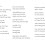how to select a particular row and particulat column
say i want 5th row and 6th column cell value?

1.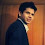income.iloc[4,5]

11.Excellent, Thanks for sharing!!!

12.A big time saver for the beginners!!! Thanks alot!!

13.hi, thanks for sharing your knowledge and congrats for the website.
just a friendly suggestion to align to the other tutorials - i think it can be added further - specifically; concatenate rows, equivalent of index/find in sas, equivalent of dcast in r, merging on non common variables, more on excel, unzip/zip, delete/copy/rename datasets..

14.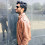Nice overview of..Pandas

15.16.Really a wonderful tutorial!

17.Very useful resource to learn Pandas..

(Dr. Nagesh Tripathi, Sr. Data Scientist, Boston Consulting Group)

18.19.great content at all one place for beginners. hope you continue good work. cheers

20.It was awesome!! I really appreciate it.

21.Hi Chris, thank you to share this. i am new to python and pandas frame work. i thought Excel data manipulations with pandas is very Difficult. these Example is really Awesome to understand the concept.

22.Thanks. The examples in this tutorial is really good and easily understandable

23.The examples in this tutorial is really good and easily understandable

24.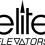It was a Nice post. Keep sharing. Home elevators | hydraulic elevators

25.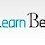This comment has been removed by the author.

26.This comment has been removed by the author.

27.Great Content! Is there any content on data visualization using Python on your website?

28.what if i want each statewise column w.r.t Y2002 so that i can predict Y2002 for each states? #timeseries

29.Thanks for sharing this information..Useful one

30.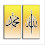This comment has been removed by a blog administrator.

31.the correct syntex for getting 6 records and 4 column rate, for an object iris,is

32.Thanks all functions covered and explained very nicely.
How can we filter the particular columns with the other one or multiple columns ?
And how to write Proc tabulate SAS procedure in Python ?

33.very useful Pandas Blog

Next → ← Prev
Looks like you are using an ad blocker!

To continue reading you need to turnoff adblocker and refresh the page. We rely on advertising to help fund our site. Please whitelist us if you enjoy our content.